# Rigid Transformations: Reflections Lesson Plan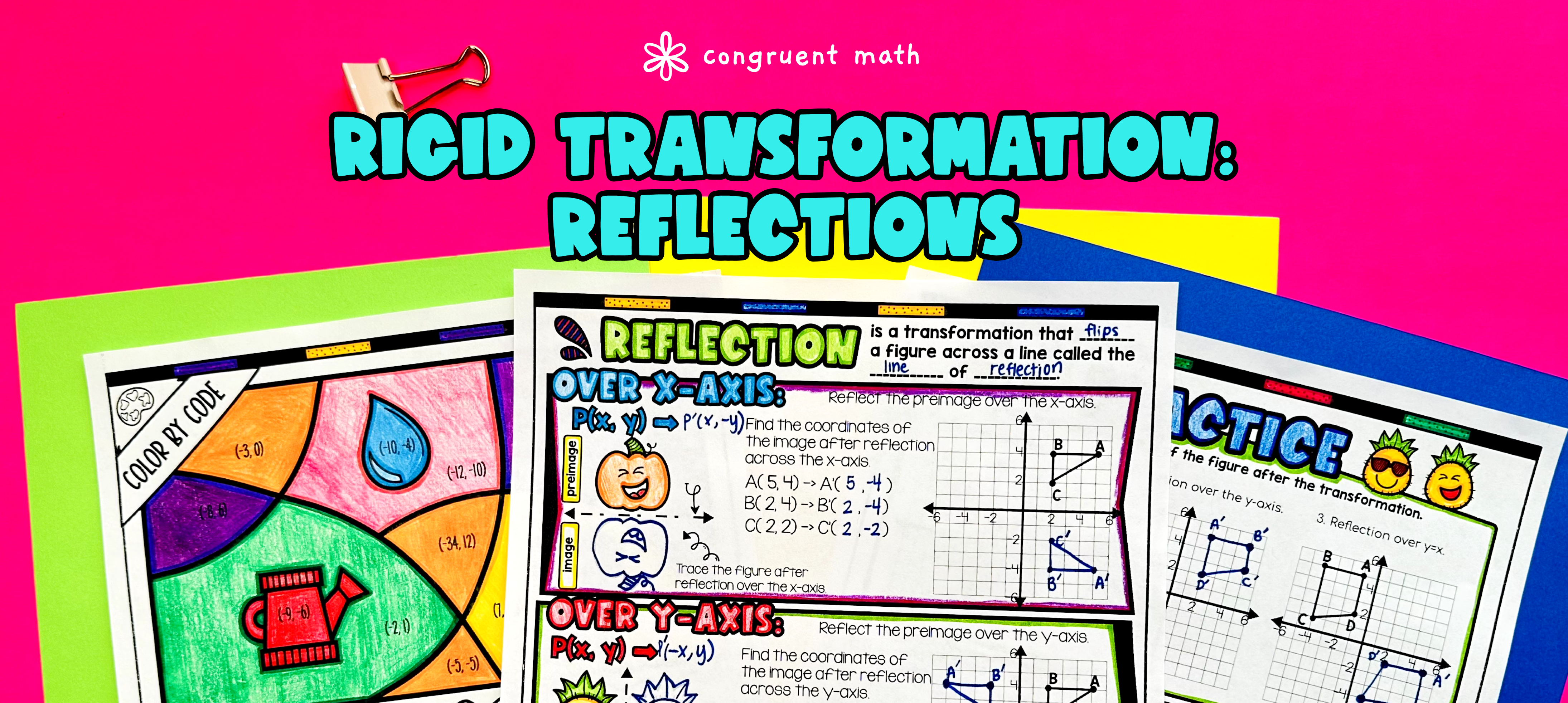Pin This

## Overview

Ever wondered how to teach rigid transformations reflections in an engaging way to your eighth-grade students?

In this lesson plan, students will learn about reflections across different axes and lines, such as the x-axis, y-axis, and the line y=x, and their real-life applications. Through artistic and interactive guided notes, structured practice activities, and a real-life application task, students will gain a comprehensive understanding of reflections.

The lesson begins with guided notes that provide structured explanations and examples of how to graph figures on coordinate planes after reflections, rules for reflections across the x-axis, y-axis, and y=x, and how to write coordinate points for preimages and images of figures undergoing reflections. These notes also include checks for understanding to ensure students are grasping the concepts.

Following the guided notes, students will engage in practice activities, including a doodle and color by number activity, a maze worksheet, and problem sets. These activities allow students to apply their understanding of reflections in a creative and interactive way, keeping them engaged and motivated.

To further connect reflections to real-life scenarios, the lesson culminates with a real-life application task. This task prompts students to read and write about real-life uses of reflections, helping them see the practical relevance of the topic beyond the classroom.

## Get the Lesson Materials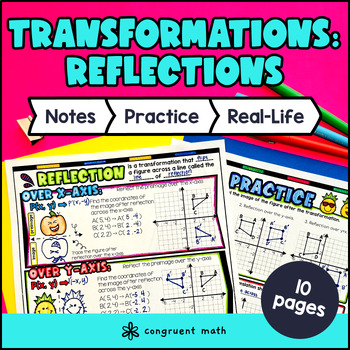\$4.25

## Learning Objectives

After this lesson, students will be able to:

• Graph figures on coordinate planes after reflections over the x-axis, y-axis, and the line y=x

• Write rules for reflections across the x-axis, y-axis, and the line y=x

• Write coordinate points for preimages and images for figures undergoing reflections over the x-axis, y-axis, and the line y=x

• Apply the concept of reflections to real-life situations and explain their significance

## Prerequisites

Before this lesson, students should be familiar with:

• Graphing points on a coordinate plane

• Identifying the x-coordinate and y-coordinate of a point

• Knowledge of basic algebraic expressions and equations (optional, but helpful)

## Key Vocabulary

• Rigid transformation

• Reflection

• X-axis

• Y-axis

• Line y=x

• Coordinate plane

• Preimage

• Image

## Procedure

### Introduction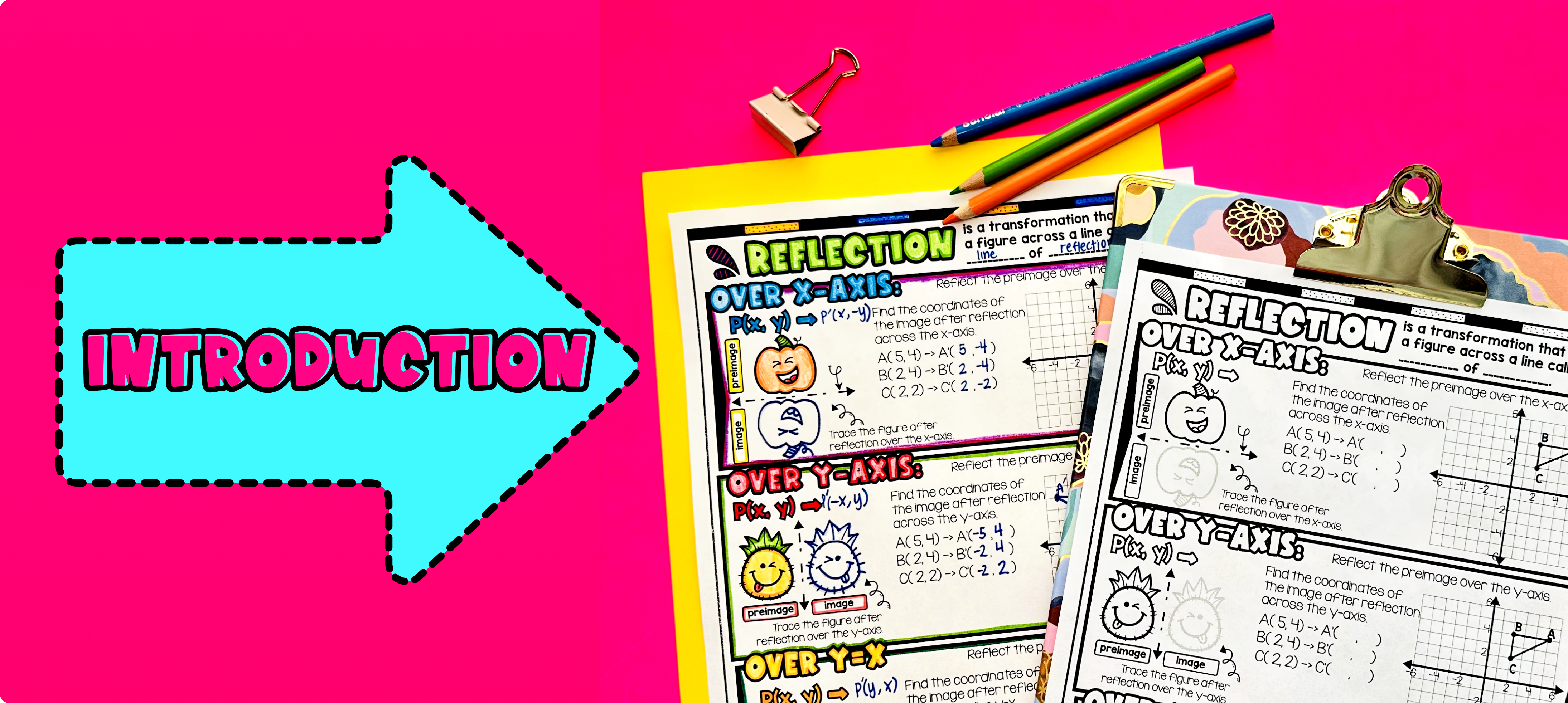Pin This

As a hook, ask students why reflections are important in real life. For example, you can ask them how reflections are used in mirror images or in designing symmetrical art pieces.

Use the first page of the guided notes to introduce the concept of rigid transformations. Have students visualize the concept by having them trace reflections of fun objects, pumpkin, emoji, and triangles, across different lines of reflections. Walk through the key points of the topic, such as identifying the line of reflection and understanding how the preimage and image are related.

Then, following the guided notes, start introducing the rules of reflections across the x-axis, y-axis and the line y=x. Help students visualize the relationship between the preimage and image, and explain how the line of reflection changes the coordinates of the points.

Have students practice using the examples on the second page of the guided notes.

Based on student responses, reteach concepts that students need extra help with. If your class has a wide range of proficiency levels, you can pull out students for reteaching and have more advanced students begin work on the practice exercises.

### Practice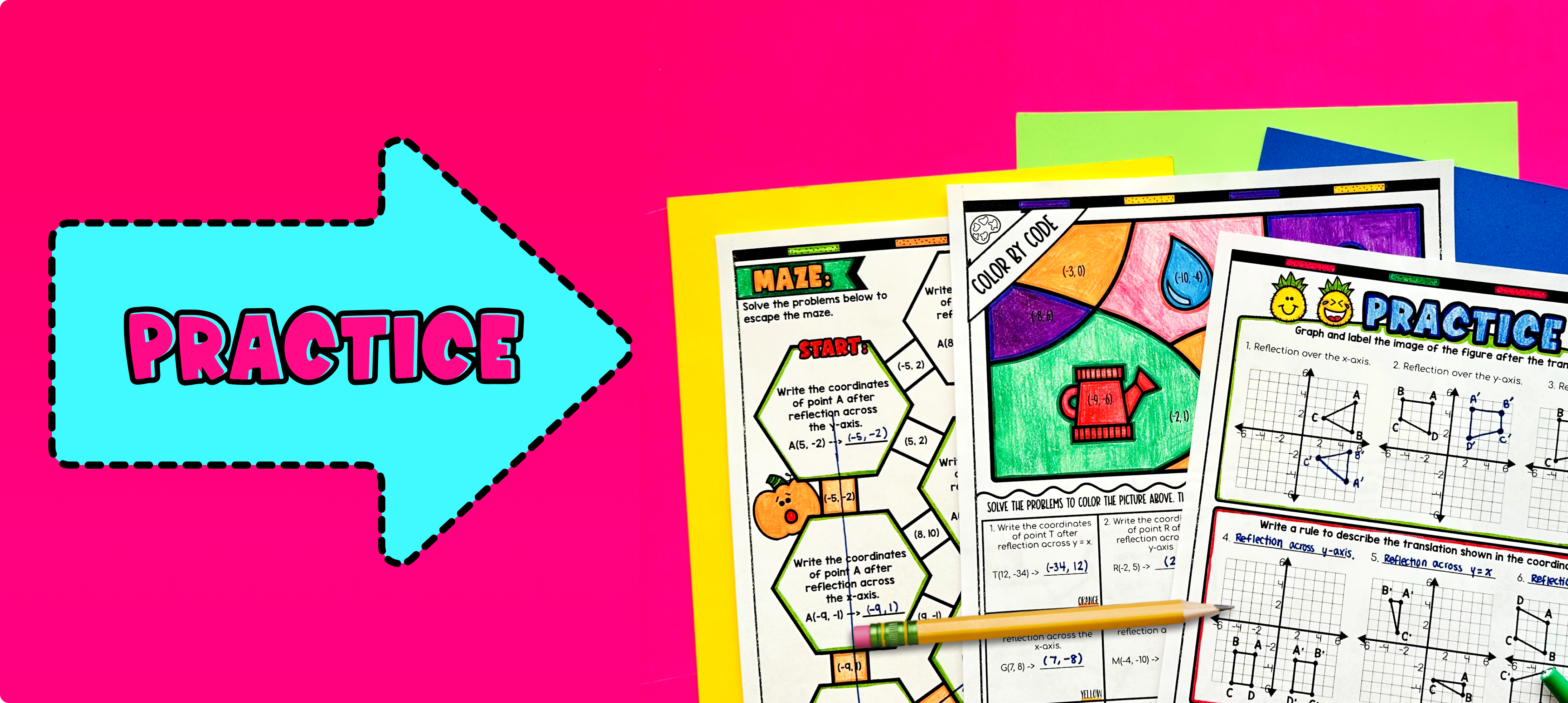Pin This

Have students practice reflection examples using the maze on the third page of the guided notes. Walk around to answer student questions.

Fast finishers can dive into the color by number activity, the 4th page of the guided notes, for extra practice. You can assign it as homework for the remainder of the class.

### Real-Life Application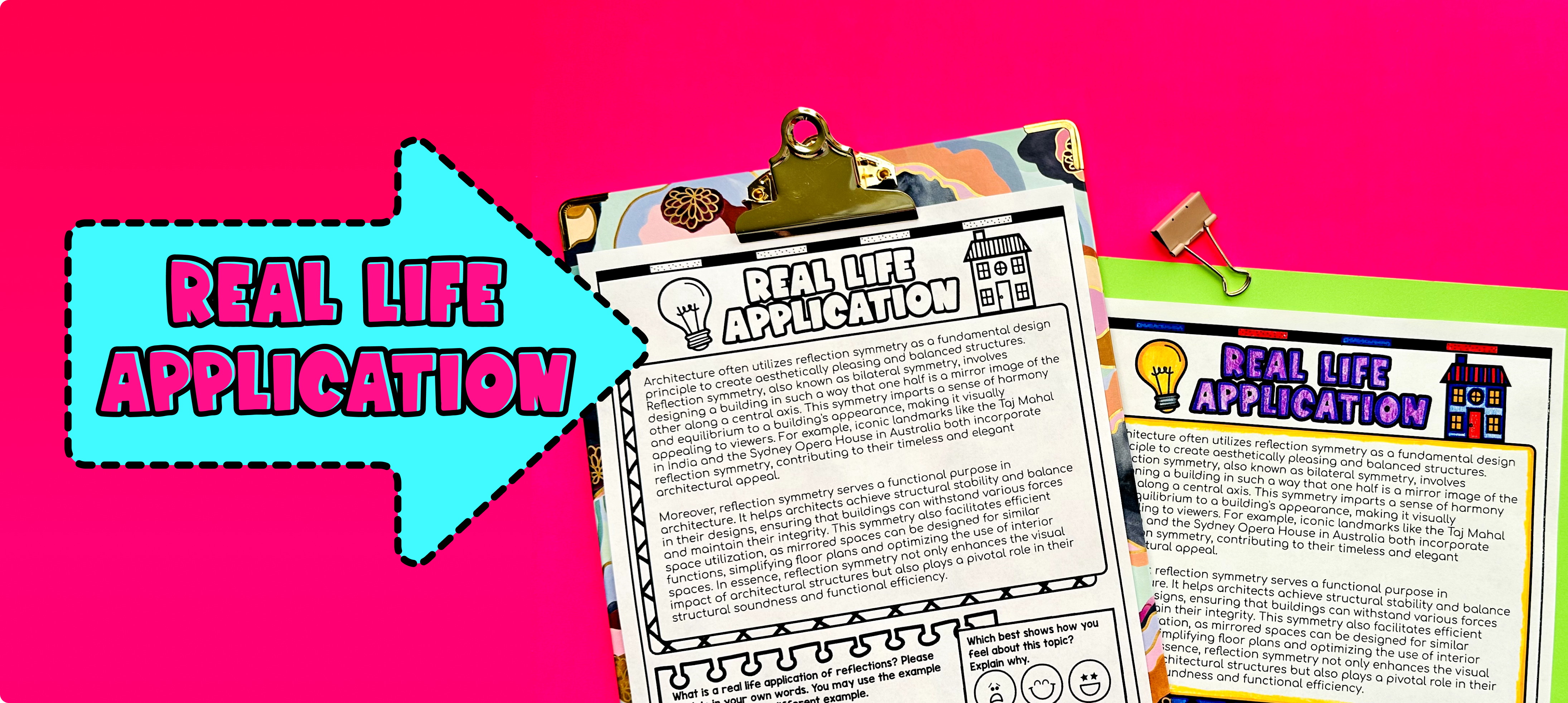Pin This

Bring the class back together, and introduce the concept of real-world applications of reflections. Explain that reflections can be seen in many different aspects of our daily lives, from mirrors to water surfaces to architecture. Use the last page of the guided notes to have students read through a detailed examples of how reflections are used in architecture.

Encourage students to share their own experiences and examples of reflections they have encountered in their lives.

Having discussed the real-life applications, you can now move on to the next activity and allow students to apply their understanding of reflections through graphing on coordinate planes.

## Extensions

If you’re looking for digital practice for reflections, try my Pixel Art activities in Google Sheets. Every answer is automatically checked, and correct answers unlock parts of a mystery picture. It’s incredibly fun, and a powerful tool for differentiation.

Here’s a reflection activity to explore: